# Linear Equations in two variables-NCERT Solutions

CHAPTER 3: PAIR OF LINEAR EQUATIONS IN TWO VARIABLES MATH
NCERT Solutions for Class 10th:
Exercise 3.1
1.       Aftab tells his daughter, “Seven years ago, I was seven times as old as you were then. Also, three years from now, I shall be three times as old as you will be.” (Isn’t this interesting?) Represent this situation algebraically and graphically.
Ans. Let the present age of Aftab = x
And, present age of his daughter is represented as = y
Seven years ago,
Aftab’s age = x – 7
Age of Aftab’s daughter = y – 7
According to the question,
(x – 7) = 7 (y – 7 )
x – 7 = 7 y – 49
x – 7y = – 49 + 7
x – 7y = – 42 …           (i)
x = 7y – 42
Putting y = 5, 6 and 7, we get
x = 7 × 5 – 42 = 35 – 42 = – 7
x = 7 × 6 – 42 = 42 – 42 = 0
x = 7 × 7 – 42 = 49 – 42 = 7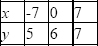Three years from now,
Aftab’s age = x + 3
Age of Aftab’s daughter = y + 3
According to the question,
(x + 3) = 3 (y + 3)
x + 3 = 3y + 9
x – 3y = 9 – 3
x – 3y = 6 …           (ii)
x = 3y + 6
Putting, y = – 2, –1 and 0, we get
x = 3 × – 2 + 6 = – 6 + 6 =0
x = 3 × – 1 + 6 = – 3 + 6 = 3
x = 3 × 0 + 6 = 0 + 6 = 6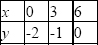Algebraic representation
From equation (i) and (ii)
x – 7y = – 42 …           (i)
x – 3y = 6 …                  (ii)
Graphical representation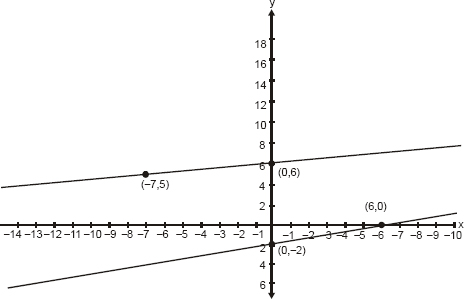2.       The coach of a cricket team buys 3 bats and 6 balls for Rs 3900. Later, she buys another bat and 3 more balls of the same kind for Rs 1300. Represent this situation algebraically and geometrically.
Ans. Let cost of one bat be = Rs x
And, cost of one ball be = Rs y
3 bats and 6 balls are for Rs 3900
Therefore, 3x + 6y = 3900 …           (i)
Dividing equation by 3, we get
x + 2y = 1300
Subtracting 2y from both side we get
x = 1300 – 2y
Putting y = –1300, 0 and 1300 we get
x = 1300 – 2 (–1300) = 1300 + 2600 = 3900
x = 1300 – 2(0) = 1300 – 0 = 1300
x = 1300 – 2(1300) = 1300 – 2600 = – 1300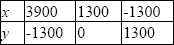Given that she buys another bat and 2 more balls of the same kind for Rs 1300
We get
x + 2y = 1300 …           (ii)
Subtracting 2y from both sides we get
x = 1300 – 2y
Putting y = – 1300, 0 and 1300 we get
x = 1300 – 2 (–1300) = 1300 + 2600 = 3900
x = 1300 – 2 (0) = 1300 – 0 = 1300
x = 1300 – 2(1300) = 1300 – 2600 = – 1300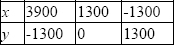Algebraic representation
3x + 6y = 3900 …           (i)
x + 2y = 1300 …              (ii)
Graphical representation,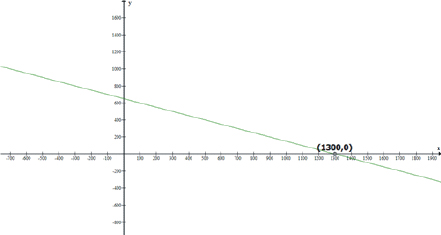3.       The cost of 2 kg of apples and 1kg of grapes on a day was found to be Rs 160. After a month, the cost of 4 kg of apples and 2 kg of grapes is Rs 300. Represent the situation algebraically and geometrically.
Ans. Let each kg of apples cost = Rs x
And, cost of each kg of grapes = Rs y
Given that the cost of 2 kg of apples and 1kg of grapes on a day was found to be = Rs 160
Therefore,
2 x + y = 160 …           (i)
2x = 160 – y
x = (160 – y)/2
Let y = 0 , 80 and 160, we get
x = (160 – ( 0 )/2 = 80
x = (160– 80 )/2 = 40
x = (160 – 2 × 80)/2 = 0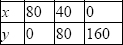Given that the cost of 4 kg of apples and 2 kg of grapes is Rs 300
Therefore,
4x + 2y = 300 …           (ii)
Dividing by 2 we get
2x + y = 150
Subtracting 2x from both the sides, we get
y = 150 – 2x
Putting x = 0 , 50 , 100 we get
y = 150 – 2 × 0 = 150
y = 150 – 2 × 50 = 50
y = 150 – 2 × (100) = – 50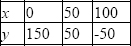Algebraic representation,
2x + y = 160 …              (i)
4x + 2y = 300 …           (ii)
Graphical representation,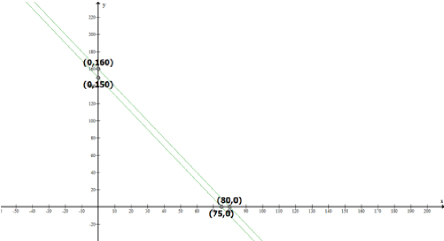Exercise 3.2
1.       Form the pair of linear equations in the following problems, and find their solutions graphically.
(i)   10 students of Class X took part in a Mathematics quiz. If the number of girls is 4 more than the number of boys, find the number of boys and girls who took part in the quiz.
Ans.    Let the number of boys be = x
Let the number of girls be = y
Given that total number of students is 10
Therefore x + y = 10
Subtracting y from both the sides we get
x = 10 – y
Putting y = 0, 5, 10 we get
x = 10 – 0 = 10
x = 10 – 5 = 5
x = 10 – 10 = 0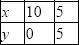Given: the number of girls is 4 more than the number of boys
Therefore
y = x + 4
Putting x = – 4, 0, 4, and we get
y = – 4 + 4 = 0
y = 0 + 4 = 4
y = 4 + 4 = 8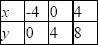Graphical representation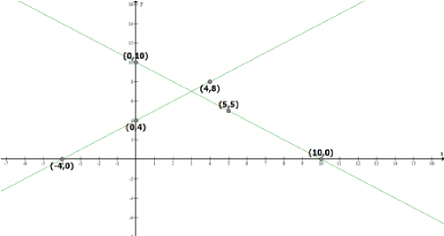Therefore, number of boys = 3 and number of girls = 7.
(ii)  5 pencils and 7 pens together cost Rs 50, whereas 7 pencils and 5 pens together cost Rs 46. Find the cost of one pencil and that of one pen.
Ans.    Let the cost of one pencil = Rs x
Let the cost of one pen = Rs y
5 pencils and 7 pens together cost = Rs 50,
Therefore
5x + 7y = 50
Subtracting 7y from both the sides we get
5x = 50 – 7y
Dividing by 5 we get
x = 10 – 7 y /5
Putting value of y = 5 , 10 and 15 we get
x = 10 – 7 × 5/5 = 10 – 7 = 3
x = 10 – 7 × 10/5 = 10 – 14 = – 4
x = 10 – 7 × 15/5 = 10 – 21 = – 11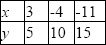Given: 7 pencils and 5 pens together cost Rs 46
7x + 5y = 46
Subtracting 7x from both the sides we get
5y = 46 – 7x
Dividing by 5 we get
y = 46/5 – 7x/5
y = 9.2 – 1.4x
Putting x = 0 , 2 and 4 we get
y = 9.2 – 1.4 × 0 = 9.2 – 0 = 9.2
y = 9.2 – 1.4 (2) = 9.2 – 2.8 = 6.4
y = 9.2 – 1.4 (4) = 9.2 – 5.6 = 3.6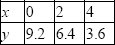Graphical representation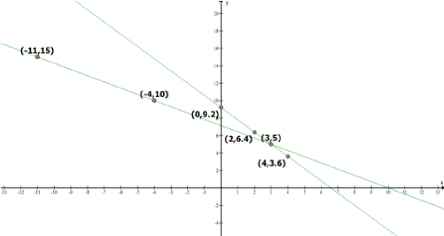Therefore, cost of one pencil = Rs 3 and cost of one pen = Rs 5.
2.       On comparing the ratios a1/a2, b1/b2 and c1/c2, find out whether the lines representing the following pairs of linear equations intersect at a point, are parallel or coincident.
Ans. (i)   5x – 4y + 8 = 0
7x + 6y – 9 = 0
On comparing these equation with
a1x + b1y + c1 = 0
a2x + b2y + c2= 0
We get
a1 = 5, b1 = – 4, and c1 = 8
a2 =7, b2 = 6 and c2 = – 9
a1/a2 = 5/7,
b1/b2 = – 4/6 and
c1/c2 = 8/–9
Hence, a1/a2 ≠ b1/b2
Therefore, both the lines intersect at one point.
(ii)  9x + 3y + 12 = 0
18x + 6y + 24 = 0
Comparing these equations with
a1x + b1y + c1 = 0
a2x + b2y + c2= 0
We get
a1 = 9, b1 = 3, and c1 = 12
a2 = 18, b2 = 6 and c2 = 24
a1/a2 = 9/18 = 1/2
b1/b2 = 3/6 = 1/2 and
c1/c2 = 12/24 = 1/2
Hence, a1/a2 = b1/b2 = c1/c2
Therefore, both the lines are coincident
(iii)  6x – 3y + 10 = 0
2x – y + 9 = 0
Comparing these equations with
a1x + b1y + c1 = 0
a2x + b2y + c2= 0
We get
a1 = 6, b1 = – 3, and c1 = 10
a2 = 2, b2 = – 1 and c2 = 9
a1/a2 = 6/2 = 3/1
b1/b2 = – 3/–1 = 3/1 and
c1/c2 = 12/24 = 1/2
Hence, a1/a2 = b1/b2 ≠ c1/c2
Therefore, both lines are parallel
3.       On comparing the ratios a1/a2, b1/b2 and c1/c2 find out whether the following pair of linear equations are consistent, or inconsistent.
(i)    3x + 2y = 5 ; 2x – 3y = 7
(ii)   2x – 3y = 8 ; 4x – 6y = 9
(iii)  3/2x + 5/3y = 7 ; 9x – 10y = 14
(iv)  5x – 3y = 11 ; – 10x + 6y = –22
(v)   4/3x + 2y =8 ; 2x + 3y = 12
Ans. (i)   3x + 2y = 5 ; 2x – 3y = 7
a1/a2 = 3/2
b1/b2 = – 2/3 and
c1/c2 = 5/7
Hence, a1/a2 ≠ b1/b2
These linear equations intersect each other at one point and therefore have only one possible solution. Hence, the pair of linear equations is consistent.
(ii)  2x – 3y = 8; 4x – 6y = 9
a1/a2 = 2/4 = 1/2
b1/b2 = – 3/–6 = 1/2 and
c1/c2 = 8/9
Hence, a1/a2 = b1/b2 ≠ c1/c2
Therefore, these linear equations are parallel to each other and thus have no possible solution.
Hence, the pair of linear equations is inconsistent.
(iii) 3/2x + 5/3y = 7 ; 9x – 10y = 14
a1/a2 = 3/2/9 = 1/6
b1/b2 = 5/3/–10 = – 1/6 and
c1/c2 = 7/14 = 1/2
Hence, a1/a2 ≠ b1/b2
Therefore, these linear equations intersect each other at one point and thus have only one possible solution. Hence, the pair of linear equations is consistent.
(iv) 5x – 3y = 11 ; – 10x + 6y = –22
a1/a2 = 5/–10 = – 1/2
b1/b2 = – 3/6 = – 1/2 and
c1/c2 = 11/–22 = – 1/2
Hence, a1/a2 = b1/b2 = c1/c2
Therefore, these linear equations are coincident and have infinite number of possible solutions.
Therefore, the pair of linear equations is consistent.
(v) 4/3x + 2y =8; 2x + 3y = 12
a1/a2 = 4/3/2 = 2/3
b1/b2 = 2 /3 and
c1/c2 = 8/12 = 2/3
Hence, a1/a2 = b1/b2 = c1/c2
Therefore, these linear equations are coincident and have infinite number of possible solutions.
Therefore, the pair of linear equations is consistent.
4.       Which of the following pairs of linear equations are consistent/inconsistent? If consistent, obtain the solution graphically:
(i)    x + y = 5, 2x + 2y = 10
(ii)   x – y = 8, 3x – 3y = 16
(iii)  2x + y – 6 = 0, 4x – 2y – 4 = 0
(iv)  2x – 2y – 2 = 0, 4x – 4y – 5 = 0
Ans. (i)   x + y = 5; 2x + 2y = 10
a1/a2 = 1/2
b1/b2 = 1/2 and
c1/c2 = 5/10 = 1/2
Hence, a1/a2 = b1/b2 = c1/c2
Therefore, these linear equations are coincident and have infinite number of possible solutions.
Therefore, the pair of linear equations is consistent.
x + y = 5
x = 5 – y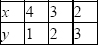And, 2x + 2y = 10
x = 10 – 2y/2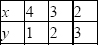Graphical representation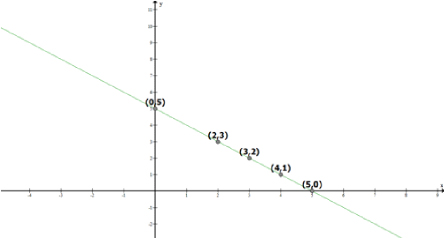From the figure, it can be observed that these lines are overlapping each other. Therefore, infinite number of solutions are possible for the given pair of equations.
(ii)  x – y = 8, 3x – 3y = 16
a1/a2 = 1/3
b1/b2 = – 1/–3 = 1/3 and
c1/c2 = 8/16 = 1/2
Hence, a1/a2 = b1/b2 ≠ c1/c2
Therefore, these linear equations are parallel to each other and thus have no possible solution.
Hence, the pair of linear equations is inconsistent.
(iii) 2x + y – 6 = 0, 4x – 2y – 4 = 0
a1/a2 = 2/4 = 1/2
b1/b2 = – 1/2 and
c1/c2 = – 6/–4 = 3/2
Hence, a1/a2 ≠ b1/b2
Therefore, these linear equations are intersecting each other at one point and thus have only one possible solution. Hence, the pair of linear equations is consistent.
2x + y – 6 = 0
y = 6 – 2x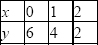And, 4x – 2y – 4 = 0
y = 4x – 4/2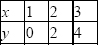Graphical representation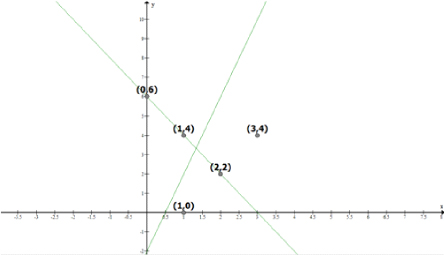From the figure, it can be observed that these lines are intersecting each other at the only one point i.e., (2,2) which is the solution for the given pair of equations.
(iv)  2x – 2y – 2 = 0, 4x – 4y – 5 = 0
a1/a2 = 2/4 = 1/2
b1/b2 = – 2/–4 = 1/2 and
c1/c2 = 2/5
Hence, a1/a2 = b1/b2 ≠ c1/c2
Therefore, these linear equations are parallel to each other and thus, have no possible solution.
Hence, the pair of linear equations is inconsistent.
5.       Half the perimeter of a rectangular garden, whose length is 4 m more than its width, is 36 m. Find the dimensions of the garden.
Ans. Let length of the rectangle be = x m
Let Width of the rectangle be = y m
According to the question,
y – x = 4 ...              (i)
y + x = 36 ...           (ii)
y – x = 4
y = x + 4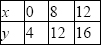y + x = 36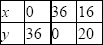Graphical representation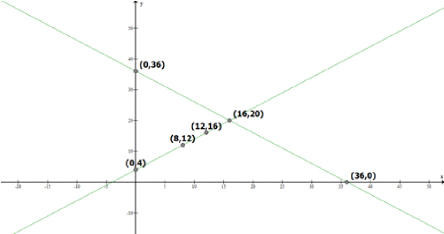From the figure, it can be seen that these lines intersect each other at only one point i.e., (16, 20).
Therefore, the length and width of the given garden is 20 m and 16 m respectively.
6.       Given the linear equation 2x + 3y – 8 = 0, write another linear equation in two variables such that the geometrical representation of the pair so formed is:
(i)    intersecting lines
(ii)   parallel lines
(iii)  coincident lines
Ans. (i)   Intersecting lines:
Condition,
a1/a2 ≠ b1/b2
The second line such that it is intersecting the given line is
2x + 4y – 6 = 0 as
a1/a2 = 2/2 = 1
b1/b2 = 3/4 and
a1/a2 ≠ b1/b2
(ii)  Parallel lines
Condition,
a1/a2 = b1/b2 ≠ c1/c2
Hence, the second line can be
4x + 6y – 8 = 0 as
a1/a2 = 2/4 = 1/2
b1/b2 = 3/6 = 1/2 and
c1/c2 = – 8/–8 = 1
and a1/a2 = b1/b2 ≠ c1/c2
(iii) Coincident lines
Condition,
a1/a2 = b1/b2 = c1/c2
Hence, the second line can be
6x + 9y – 24 = 0 as
a1/a2 = 2/6 = 1/3
b1/b2 = 3/9 = 1/3 and
c1/c2 = – 8/–24 = 1/3
and a1/a2 = b1/b2 = c1/c2
7.       Draw the graphs of the equations x – y + 1 = 0 and 3x + 2y – 12 = 0. Determine the coordinates of the vertices of the triangle formed by these lines and the x-axis, and shade the triangular region.
Ans. x – y + 1 = 0
x = y – 1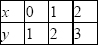3x + 2y – 12 = 0
x = 12 – 2y/3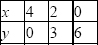Graphical representation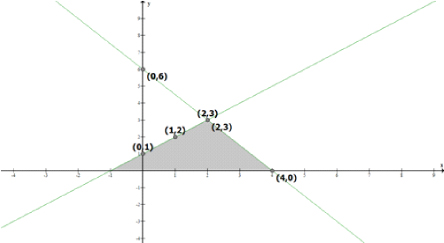From the figure, it can be seen that these lines are intersecting each other at point (2, 3) and x-axis at ( – 1, 0) and (4, 0). Therefore, the vertices of the triangle are (2, 3), ( – 1, 0), and (4, 0).
Exercise 3.3
1.       Solve the following pair of linear equations by the substitution method.
(i)    x + y = 14 ; x – y = 4
(ii)   s – t = 3 ; s/3 + t/2 = 6
(iii)  3x – y = 3 ; 9x – 3y = 9
(iv)  0.2x + 0.3y = 1.3 ; 0.4x + 0.5y = 2.3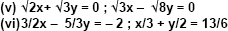Ans. (i)   x + y = 14 ... (i)
x – y = 4 ... (ii)
From equation (i), we get
x = 14 – y ... (iii)
Putting this value in equation (ii), we get
(14 – y) – y = 4
14 – 2y = 4
10 = 2y
y = 5 ... (iv)
Putting this in equation (iii), we get
x = 9
∴ x = 9 and y = 5
(ii)  s – t = 3 ... (i)
s/3 + t/2 = 6 ... (ii)
From equation (i), we get s = t + 3
Putting this value in equation (ii), we get
t + 3/3 + t/2 = 6
2t + 6 + 3t = 36
5t = 30
t = 30/5 ... (iv)
Putting in equation (iii), we get
s = 9
∴ s = 9, t = 6
(iii) 3x – y = 3 ... (i)
9x – 3y = 9 ... (ii)
From equation (i), we get
y = 3x – 3 ... (iii)
Putting this value in equation (ii), we get
9x – 3(3x – 3) = 9
9x – 9x + 9 = 9
9 = 9
This is always true.
Hence, the given pair of equations has infinite possible solutions and the relation between these variables can be given by y = 3x – 3
Therefore, one of its possible solutions is x = 1, y = 0.
(iv) 0.2x + 0.3y = 1.3 ... (i)
0.4x + 0.5y = 2.3 ... (ii)
0.2x + 0.3y = 1.3
Solving equation (i), we get
0.2x = 1.3 – 0.3y
Dividing by 0.2, we get
x = 1.3/0.2 – 0.3/0.2
x = 6.5 – 1.5 y ... (iii)
Putting the value in equation (ii), we get
0.4x + 0.5y = 2.3
(6.5 – 1.5y) × 0.4x + 0.5y = 2.3
2.6 – 0.6y + 0.5y = 2.3
–0.1y = 2.3 – 2.6
y = – 0.3/–0.1
y = 3
Putting this value in equation (iii) we get
x = 6.5 – 1.5 y
x = 6.5 – 1.5(3)
x = 6.5 – 4.5
x = 2
∴ x = 2 and y = 3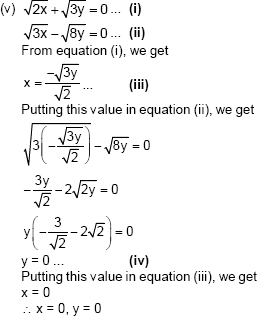(vi) 3/2x – 5/3y = – 2 ... (i)
x/3 + y/2 = 13/6 ... (ii)
From equation (i), we get
9x – 10y = – 12
x = – 12 + 10y/9 ... (iii)
Putting this value in equation (ii), we get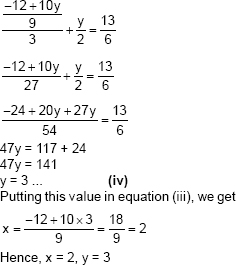2.       Solve 2x + 3y = 11 and 2x – 4y = – 24 and hence find the value of ‘m’ for which y = mx + 3.
Ans. 2x + 3y = 11 ... (i)
Subtracting 3y both side we get
2x = 11 – 3y … (ii)
Putting this value in equation second we get
2x – 4y = – 24 … (iii)
11- 3y – 4y = – 24
7y = – 24 – 11
–7y = – 35
y = – 35/–7
y = 5
Putting this value in equation (iii) we get
2x = 11 – 3 × 5
2x = 11 – 15
2x = – 4
Dividing by 2 we get
x = – 2
Putting the value of x and y
y = mx + 3.
5 = – 2m + 3
2m = 3 – 5
m = – 2/2
m = – 1
3.       Form the pair of linear equations for the following problems and find their solution by substitution method.
(i)    The difference between two numbers is 26 and one number is three times the other.
Find them.
Ans. Let the larger number be = x
Let the smaller number be = y
The difference between the two numbers is 26
x – y = 26
x = 26 + y
Given that one number is three times the other
So x = 3y
Putting the value of x we get
26 + y = 3y
–2y = – 2 6
y = 13
So value of x = 3y
Putting value of y, we get
x = 3 × 13 = 39
Therefore the numbers are 13 and 39.
(ii)  The larger of two supplementary angles exceeds the smaller by 18 degrees. Find them.
Ans. Let the first angle be= x
And the second angle be = y
As both angles are supplementary so that sum will 180
x + y = 180
x = 180 – y ... (i)
Given; difference is 18 degree
Therefore
x – y = 18
Putting the value of x we get
180 – y – y = 18
– 2y = – 162
y = – 162/–2
y = 81
Putting the value back in equation (i), we get
x = 180 – 81 = 99 Hence, the angles are 99° and 81°.
(iii) The coach of a cricket team buys 7 bats and 6 balls for Rs 3800. Later, she buys 3 bats
and 5 balls for Rs 1750. Find the cost of each bat and each ball.
Ans. Let the cost of each bat be = Rs x
Let the cost of each ball be = Rs y
Given: coach of a cricket team buys 7 bats and 6 balls for Rs 3800.
7x + 6y = 3800
6y = 3800 – 7x
Dividing by 6, we get
y = (3800 – 7x)/6 … (i)
Given that she buys 3 bats and 5 balls for Rs 1750 later.
3x + 5y = 1750
Putting the value of y
3x + 5 ((3800 – 7x)/6) = 1750
Multiplying by 6, we get
18x + 19000 – 35x = 10500
–17x =10500 – 19000
–17x = – 8500
x = – 8500/–17
x = 500
Putting this value in equation (i) we get
y = ( 3800 – 7 × 500)/6
y = 300/6
y = 50
Hence the cost of each bat = Rs 500 and the cost of each balls = Rs 50.
(iv) The taxi charges in a city consist of a fixed charge together with the charge for the distance covered. For a distance of 10 km, the charge paid is Rs 105 and for a journey of 15 km, the charge paid is Rs 155. What are the fixed charges and the charge per km?
How much does a person have to pay for traveling a distance of 25 km?
Ans. Let the fixed charge for taxi = Rs x
And variable cost per km = Rs y
Total cost = fixed charge + variable charge
Given that for a distance of 10 km, the charge paid is Rs 105
x + 10y = 105 … (i)
x = 105 – 10y
Given that for a journey of 15 km, the charge paid is Rs 155
x + 15y = 155
Putting the value of x we get
105 – 10y + 15y = 155
5y = 155 – 105
5y = 50
Dividing by 5, we get
y = 50/5 = 10
Putting this value in equation (i) we get
x = 105 – 10 × 10
x = 5
Cost for traveling a distance of 25 km
= x + 25y
= 5 + 25 × 10
= 5 + 250
=255
A person has to pay Rs 255 for 25 Km.
(v)  A fraction becomes 9/11, if 2 is added to both the numerator and the denominator. If, 3 is added to both the numerator and the denominator it becomes 5/6. Find the fraction.
Ans. Let the Numerator be = x
Let the Denominator be = y
Fraction = x/y
A fraction becomes 9/11, if 2 is added to both the numerator and the denominator
(x + 2)/y + 2 = 9/11
On Cross multiplying,
11x + 22 = 9y + 18
Subtracting 22 from both sides,
11x = 9y – 4
Dividing by 11, we get
x = 9y – 4/11 … (i)
Given: if 3 is added to both the numerator and the denominator it becomes 5/6.
(x+3)/y +3 = 5/6 … (ii)
On Cross multiplying,
6x + 18 = 5y + 15
Subtracting the value of x, we get
6(9y – 4 )/11 + 18 = 5y + 15
Subtracting 18 from both the sides
6(9y – 4 )/11 = 5y – 3
54 – 24 = 55y – 33
–y = – 9
y = 9
Putting this value of y in equation (i), we get
x = 9y – 4
11 … (i)
x = (81 – 4)/77
x = 77/11
x = 7
Hence our fraction is 7/9.
(vi) Five years hence, the age of Jacob will be three times that of his son. Five years ago, Jacob’s age was seven times that of his son. What are their present ages?
Ans. Let the present age of Jacob be = x year
And the present Age of his son be = y year
Five years from now,
Jacob’s age will be = x + 5 year
Age of his son will be = y + 5year
Given; the age of Jacob will be three times that of his son
x + 5 = 3(y + 5)
x = 3y + 15 – 5
x = 3y + 10 … (i)
Five years ago,
Jacob’s age= x – 5 year
His son’s age = y – 5 year
Jacob’s age was seven times that of his son
x – 5 = 7(y – 5)
Putting the value of x from equation (i) we get
3y + 10 – 5 = 7y – 35
3y + 5 = 7y – 35
3y – 7y = – 35 – 5
–4y = – 40
y = – 40/–4
y = 10 year
Putting the value of y in equation we get
x = 3 × 10 + 10
x = 40 years
Hence, Present age of Jacob = 40 years and present age of his son = 10 years.
Exercise 3.4
1.       Solve the following pair of linear equations by the elimination method and the substitution method:
(i)    x + y =5 and 2x –3y = 4
(ii)   3x + 4y = 10 and 2x – 2y = 2
(iii)  3x – 5y – 4 = 0 and 9x = 2y + 7
(iv)  x/2 + 2y/3 = – 1 and x – y/3 = 3
Ans. (i) x + y =5 and 2x –3y = 4
By elimination method
x + y =5 ... (i)
2x –3y = 4 ... (ii)
Multiplying equation (i) by (ii), we get
2x + 2y = 10 ... (iii)
2x – 3y = 4 ... (ii)
Subtracting equation (ii) from equation (iii), we get
5y = 6
y = 6/5
Putting the value in equation (i), we get
x = 5 – (6/5) = 19/5
Hence, x = 19/5 and y = 6/5
By substitution method
x + y = 5 ... (i)
Subtracting y from both side, we get
x = 5 – y ... (iv)
Putting the value of x in equation (ii) we get
2(5 – y) – 3y = 4
–5y = – 6
y = – 6/–5 = 6/5
Putting the value of y in equation (iv) we get
x = 5 – 6/5
x = 19/5
Hence, x = 19/5 and y = 6/5 again
(ii)  3x + 4y = 10 and 2x – 2y = 2
By elimination method
3x + 4y = 10 .... (i)
2x – 2y = 2 ... (ii)
Multiplying equation (ii) by 2, we get
4x – 4y = 4 ... (iii)
3x + 4y = 10 ... (i)
Adding equation (i) and (iii), we get
7x + 0 = 14
Dividing both side by 7, we get
x = 14/7 = 2
Putting in equation (i), we get
3x + 4y = 10
3(2) + 4y = 10
6 + 4y = 10
4y = 10 – 6
4y = 4
y = 4/4 = 1
Hence, answer is x = 2, y = 1
By substitution method
3x + 4y = 10 ... (i)
Subtract 3x both side, we get
4y = 10 – 3x
Divide by 4 we get
y = (10 – 3x )/4
Putting this value in equation (ii), we get
2x – 2y = 2 ... (i)
2x – 2(10 – 3x )/4) = 2
Multiply by 4 we get
8x – 2(10 – 3x) = 8
8x – 20 + 6x = 8
14x = 28
x = 28/14 = 2
y = (10 – 3x)/4
y = 4/4 = 1
Hence, answer is x = 2, y = 1 again.
(iii) 3x – 5y – 4 = 0 and 9x = 2y + 7
By elimination method
3x – 5y – 4 = 0
3x – 5y = 4 ...(i)
9x = 2y + 7
9x – 2y = 7 ... (ii)
Multiplying equation (i) by 3, we get
9 x – 15 y = 11 ... (iii)
9x – 2y = 7 ... (ii)
Subtracting equation (ii) from equation (iii), we get
–13y = 5
y = – 5/13
Putting value in equation (i), we get
3x – 5y = 4 ... (i)
3x – 5(–5/13) = 4
Multiplying by 13 we get
39x + 25 = 52
39x = 27
x =27/39 = 9/13
Hence our answer is x = 9/13 and y = – 5/13
By substitution method
3x – 5y = 4 ... (i)
Adding 5y on both sides we get
3x = 4 + 5y
Dividing by 3 we get
x = (4 + 5y )/3 ... (iv)
Putting this value in equation (ii) we get
9x – 2y = 7 ... (ii)
9 ((4 + 5y )/3) – 2y = 7
On solve we get
3(4 + 5y ) – 2y = 7
12 + 15y – 2y = 7
13y = – 5
y = – 5/13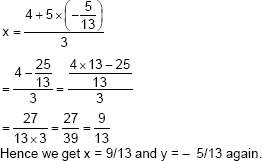(iv) x/2 + 2y/3 = – 1 and x – y/3 = 3
By elimination method
x/2 + 2y/3 = – 1 ... (i)
x – y/3 = 3 ... (ii)
Multiplying equation (i) by 2, we get
x + 4y/3 = – 2 ... (iii)
x – y/3 = 3 ... (ii)
Subtracting equation (ii) from equation (iii), we get
5y/3 = – 5
Dividing by 5 and multiplying by 3, we get
y = – 15/5
y = – 3
Putting this value in equation (ii), we get
x – y/3 = 3 ... (ii)
x – (–3)/3 = 3
x + 1 = 3
x = 2
Therefore x = 2 and y = – 3.
By substitution method
x – y/3 = 3 ... (ii)
Adding y/3 on both sides, we get
x = 3 + y/3 ... (iv)
Putting the value in equation (i) we get
x/2 + 2y/3 = – 1 ... (i)
(3+ y/3)/2 + 2y/3 = – 1
3/2 + y/6 + 2y/3 = – 1
Multiplying by 6,
9 + y + 4y = – 6
5y = – 15
y = – 3
Therefore x = 2 and y = – 3.
2.       Form the pair of linear equations in the following problems, and find their solutions (if they exist) by the elimination method:
(i)    If we add 1 to the numerator and subtract 1 from the denominator, a fraction reduces to 1. It becomes 1/2 if we only add 1 to the denominator. What is the fraction?
(ii)   Five years ago, Nuri was thrice as old as Sonu. Ten years later, Nuri will be twice as old as Sonu. How old are Nuri and Sonu?
(iii)  The sum of the digits of a two-digit number is 9. Also, nine times this number is twice the number obtained by reversing the order of the digits. Find the number.
(iv)  Meena went to bank to withdraw Rs 2000. She asked the cashier to give her Rs 50 and Rs 100 notes only. Meena got 25 notes in all. Find how many notes of Rs 50 and Rs 100 she received.
(v)   A lending library has a fixed charge for the first three days and an additional charge for each day thereafter. Saritha paid Rs 27 for a book kept for seven days, while Susy paid Rs 21 for the book she kept for five days. Find the fixed charge and the charge for each extra day.

Ans. (i) Let the fraction be x/y
According to the question, x + 1/y – 1 = 1
⇒ x – y = – 2 ... (i) x/y + 1 = 1/2
⇒ 2x – y = 1 ... (ii)
Subtracting equation (i) from equation (ii), we get
x = 3 ... (iii)
Putting this value in equation (i), we get
3 – y = – 2
–y = – 5
y = 5
Hence, the fraction is 3/5
(ii)  Let the present age of Nuri be = x
Let the present age of Sonu be = y
According to the given information,
(x – 5) = 3(y – 5)
x – 3y = – 10 ... (i)
(x + 10y) = 2(y + 10)
x – 2y = 10 ... (ii)
Subtracting equation (i) from equation (ii), we get
y = 20 ... (iii)
Putting this value in equation (i), we get
x – 60 = – 10
x = 50
Hence, age of Nuri = 50 years and age of Sonu = 20 years.
(iii) Let the unit digit and tens digits of the number be x and y respectively.
Then, number = 10y + x
Number after reversing the digits = 10x + y
According to the question,
x + y = 9 ... (i)
9(10y + x) = 2(10x + y)
88y – 11x = 0
–x + 8y =0 ... (ii)
Adding equation (i) and (ii), we get
9y = 9
y = 1 ... (iii)
Putting the value in equation (i), we get
x = 8
Hence, the number is 10y + x = 10 × 1 + 8 = 18.
(iv)  Let the number of Rs 50 notes and Rs 100 notes be x and y respectively.
According to the question,
x + y = 25 ... (i)
50x + 100y = 2000 ... (ii)
Multiplying equation (i) by 50, we get
50x + 50y = 1250 ... (iii)
Subtracting equation (iii) from equation (ii), we get
50y = 750
y = 15
Putting this value in equation (i), we have x = 10
Hence, Meena has 10 notes of Rs 50 and 15 notes of Rs 100.
(v)   Let the fixed charge for first three days and each day charge thereafter be Rs x and Rs y respectively.
According to the question,
x + 4y = 27 ... (i)
x + 2y = 21 ... (ii)
Subtracting equation (ii) from equation (i), we get
2y = 6
y = 3 ... (iii)
Putting in equation (i), we get
x + 12 =27
x = 15
Hence, fixed charge = Rs 15 and Charge per day = Rs 3.
Exercise 3.5
1.       Which of the following pairs of linear equations has unique solution, no solution or infinitely many solutions? In case there is a unique solution, find it by using cross multiplication method.
(i)    x – 3y – 3 = 0 ; 3x – 9y – 2 =0
(ii)   2x + y = 5 ; 3x + 2y = 8
(iii)  3x – 5y = 20 ; 6x – 10y = 40
(iv)  x – 3y – 7 = 0 ; 3x – 3y – 15= 0
Ans. (i) x – 3y – 3 = 0
3x – 9y – 2 =0
a1/a2 = 1/3
b1/b2 = – 3/-9 = 1/3 and
c1/c2 = – 3/-2 = 3/2
a1/a2 = b1/b2 ≠ c1/c2
Therefore, the given sets of lines are parallel to each other. Therefore, they will not intersect each other and thus, there will not be any solution for these equations.
(ii)  2x + y = 5
3x + 2y = 8
a1/a2 = 2/3
b1/b2 = 1/2 and
c1/c2 = – 5/–8 = 5/8
a1/a2 ≠ b1/b2
Therefore, they will intersect each other at a unique point and thus, there will be a unique solution for these equations.
By cross-multiplication method,
x/b1c2– b2c1 = y/c1a2 – c2a1 = 1/a1b2 – a2b1
x/-8–(–10) = y/–15 + 16 = 1/4 – 3
x/2 = y/1 = 1
x/2 = 1, y/1 = 1
∴ x = 2, y = 1.
(iii) 3x – 5y = 20
6x – 10y = 40
a1/a2 = 3/6 = 1/2
b1/b2 = – 5/–10 = 1/2 and
c1/c2 = – 20/–40 = 1/2
a1/a2 = b1/b2 = c1/c2
Therefore, the given sets of lines will be overlapping each other i.e., the lines will be coincident to each other and thus, there are infinite solutions possible for these equations.
(iv) x – 3y – 7 = 0
3x – 3y – 15= 0
a1/a2 = 1/3
b1/b2 = – 3/–3 = 1 and
c1/c2 = – 7/–15 = 7/15
a1/a2 ≠ b1/b2
Therefore, they will intersect each other at a unique point and thus, there will be a unique solution for these equations.
By cross-multiplication,
x/45 – (21) = y/–21 – (–15) = 1/–3 – (–9)
x/24 = y/–6 = 1/6
x/24 = 1/6 and y/–6 = 1/6
x = 4 and y = – 1
∴ x = 4, y = – 1.
2.       (i) For which values of a and b does the following pair of linear equations have an infinite number of solutions?
2x + 3y =7
(a – b)x + (a + b)y = 3a + b –2
Ans. 2x + 3y –7 = 0
(a – b)x + (a + b)y – (3a + b –2) = 0
a1/a2 = 2/a – b = 1/2
b1/b2 = – 7/a + b and
c1/c2 = – 7/–(3a + b – 2) = 7/(3a + b – 2)
For infinitely many solutions,a1/a2 = b1/b2 = c1/c2
2/a – b = 7/3a + b – 26a + 2b – 4 = 7a – 7b
a – 9b = – 4 ... (i)
2/a – b = 3/a+b
2a + 2b = 3a – 3b
a – 5b = 0 ... (ii)
Subtracting equation (i) from (ii), we get
4b = 4
b = 1
Putting this value in equation (ii), we get
a – 5 × 1 = 0
a = 5
Hence, a = 5 and b = 1 are the values for which the given equations give infinitely many solutions.
(ii)  For which value of k will the following pair of linear equations have no solution?
3x + y = 1
(2k –1)x + (k –1)y = 2k + 1
Ans. 3x + y –1 = 0
(2k –1)x + (k –1)y – (2k + 1) = 0
a1/a2 = 3/2k – 1
b1/b2 = 1/k – 1 and
c1/c2 = – 1/–2k – 1 = 1/2k + 1
For no solutions,
a1/a2 = b1/b2 ≠ c1/c2
3/2k – 1 = 1/k-1 ≠ 1/2k + 1
3/2k – 1 = 1/k – 1
3k – 3 = 2k – 1
k = 2
Hence, for k = 2, the given equation has no solution.
3.       Solve the following pair of linear equations by the substitution and cross-multiplication methods:
8x + 5y = 9
3x + 2y = 4
Ans. 8x + 5y = 9 ... (i)
3x + 2y = 4 ... (ii)
From equation (ii), we get
x = 4 – 2y/3 ... (iii)
Putting this value in equation (i), we get
8(4 – 2y/3) + 5y = 9
32 – 16y + 15y = 27
–y = – 5
y = 5 ... (iv)
Putting this value in equation (ii), we get
3x + 10 = 4
x = – 2
Hence, x = – 2, y = 5
By cross multiplication again, we get
8x + 5y – 9 = 0
3x + 2y – 4 = 0
x/–20 – (–18) = y/–27 – (–32) = 1/16 – 15
x/–2 = y/5 = 1/1
x/–2 = 1 and y/5 = 1
x = – 2 and y = 5
4.       orm the pair of linear equations in the following problems and find their solutions (if they exist) by any algebraic method:
(i)    A part of monthly hostel charges is fixed and the remaining depends on the number of days one has taken food in the mess. When a student A takes food for 20 days she has to pay Rs 1000 as hostel charges whereas a student B, who takes food for 26 days, pays Rs 1180 as hostel charges. Find the fixed charges and the cost of food per day.
Ans. Let x be the fixed charge of the food and y be the charge for food per day.
According to the question,
x + 20y = 1000 ... (i)
x + 26y = 1180 ... (ii)
Subtracting equation (i) from equation (ii), we get
6y = 180
y = 180/6 = 30
Putting this value in equation (i), we get
x + 20 × 30 = 1000
x = 1000 – 600
x = 400
Hence, fixed charge = Rs 400 and charge per day = Rs 30
(ii)  A fraction becomes 1/3 when 1 is subtracted from the numerator and it becomes 1/4
when 8 is added to its denominator. Find the fraction.
Ans. Let the fraction be x/y
According to the question,
x–1/y = 1/3
⇒ 3x – y = 3... (i)
x/y + 8 = 1/4
⇒ 4x – y = 8 ... (ii)
Subtracting equation (i) from equation (ii), we get
x = 5 ... (iii)
Putting this value in equation (i), we get
15 – y = 3
y = 12
Hence, the fraction is 5/12.
(iii) Yash scored 40 marks in a test, getting 3 marks for each right answer and losing 1 mark for each wrong answer. Had 4 marks been awarded for each correct answer and 2 marks been deducted for each incorrect answer, then Yash would have scored 50 marks. How many questions were there in the test?
Ans. Let the number of right answers and wrong answers be x and y respectively.
According to the question,
3x – y = 40 ... (i)
4x – 2y = 50
⇒ 2x – y = 25 ... (ii)
Subtracting equation (ii) from equation (i), we get
x = 15 ... (iii)
Putting this value in equation (ii), we get
30 – y = 25
y = 5
Therefore, number of right answers = 15
And number of wrong answers = 5
Total number of questions = 20
(iv) Places A and B are 100 km apart on a highway. One car starts from A and another from B at the same time. If the cars travel in the same direction at different speeds, they meet in 5 hours. If they travel towards each other, they meet in 1 hour. What are the speeds of the two cars?
Ans. Let the speed of 1st car and 2nd car be u km/h and v km/h.
Respective speed of both cars while they are travelling in same direction = (u – v) km/h
Respective speed of both cars while they are travelling in opposite directions i.e., travelling towards each other = (u + v) km/h
According to the question,
5(u – v) = 100
⇒ u – v = 20 ... (i)
1(u + v) = 100 ... (ii)
Adding both the equations, we get
2u = 120
u = 60 km/h ... (iii)
Putting this value in equation (ii), we obtain
v = 40 km/h
Hence, speed of one car = 60 km/h and speed of other car = 40 km/h
(v)  The area of a rectangle gets reduced by 9 square units, if its length is reduced by 5 units and breadth is increased by 3 units. If we increase the length by 3 units and the breadth by 2 units, the area increases by 67 square units. Find the dimensions of the rectangle.
Ans. Let length and breadth of rectangle be x unit and y unit respectively.
Area = xy
According to the question,
(x – 5) (y + 3) = xy – 9
⇒ 3x – 5y – 6 = 0 ... (i)
(x + 3) (y + 2) = xy + 67
⇒ 2x – 3y – 61 = 0 ... (ii)
By cross multiplication, we get
x/305 – (–18) = y/–12 – (–183) = 1/9 – (–10)
x/323 = y/171 = 1/19
x = 17, y = 9
Hence, the length of the rectangle = 17 units and breadth of the rectangle = 9 units.
Exercise 3.6
1.       Solve the following pairs of equations by reducing them to a pair of linear equations: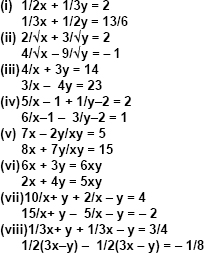Ans. (i) 1/2x + 1/3y = 2
1/3x + 1/2y = 13/6
Let 1/x = p and 1/y = q, then the equations changes as below:
p/2 + q/3 = 2
⇒ 3p + 2q – 12 = 0 ... (i)
p/3 + q/2 = 13/6
⇒ 2p + 3q – 13 = 0 ... (ii)
By cross-multiplication method, we get
p/–26 – (–36) = q/–24 – (–39) = 1/9–4
p/10 = q/15 = 1/5
p/10 = 1/5 and q/15 = 1/5
p = 2 and q = 3
1/x = 2 and 1/y = 3
Hence, x = 1/2 and y = 1/3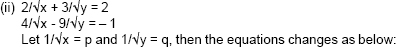2p + 3q = 2 ... (i)
4p – 9q = – 1 ... (ii)
Multiplying equation (i) by 3, we get
6p + 9q = 6 ... (iii)
Adding equation (ii) and (iii), we get
10p = 5
p = 1/2 ... (iv)
Putting in equation (i), we get
2 × 1/2 + 3q = 2
3q = 1
q = 1/3
p = 1/vx = 1/2
vx = 2
x = 4
and
q = 1/vy = 1/3
vy = 3
y = 9
Hence, x = 4, y = 9
(iii) 4/x + 3y = 14
3/x – 4y = 23
Putting 1/x = p in the given equations, we get
4p + 3y = 14
⇒ 4p + 3y – 14 = 0
3p – 4y = 23
⇒ 3p – 4y – 23 = 0
By cross-multiplication, we get
p/–69 – 56 = y/–42 – (–92) = 1/–16 – 9
⇒ – p/125 = y/50 = – 1/25
Now,
–p/125 = – 1/25 and y/50 = – 1/25
⇒ p = 5 and y = – 2
Also, p = 1/x = 5
⇒ x = 1/5
So, x = 1/5 and y = – 2 is the solution.
(iv) 5/x – 1 + 1/y – 2 = 2
6/x – 1 – 3/y – 2 = 1
Putting 1/x – 1 = p and 1/y – 2 = q in the given equations, we obtain
5p + q = 2 ... (i)
6p – 3q = 1 ... (ii)
Now, by multiplying equation (i) by 3 we get
15p + 3q = 6 ... (iii)
Now, adding equation (ii) and (iii)
21p = 7
⇒ p = 1/3
Putting this value in equation (ii) we get,
6×1/3 – 3q =1
⇒ 2 – 3q = 1
⇒ – 3q = 1 – 2
⇒ – 3q = – 1
⇒ q = 1/3
Now,
p = 1/x – 1 = 1/3
⇒1/x – 1 = 1/3
⇒ 3 = x – 1
⇒ x = 4
Also,
q = 1/y – 2 = 1/3
⇒ 1/y – 2 = 1/3
⇒ 3 = y – 2
⇒ y = 5
Hence, x = 4 and y = 5 is the solution.
(v)  7x – 2y/xy = 5
⇒ 7x/xy – 2y/xy = 5
⇒ 7/y – 2/x = 5 ... (i)
8x + 7y/xy = 15
⇒ 8x/xy + 7y/xy = 15
⇒ 8/y + 7/x = 15 ... (ii)
Putting 1/x = p and 1/y = q in (i) and (ii) we get,
7q – 2p = 5 ... (iii)
8q + 7p = 15 ... (iv)
Multiplying equation (iii) by 7 and multiplying equation (iv) by 2 we get,
49q – 14p = 35 ... (v)
16q + 14p = 30 ... (vi)
Now, adding equation (v) and (vi) we get,
49q – 14p + 16q + 14p = 35 + 30
⇒ 65q = 65
⇒ q = 1
Putting the value of q in equation (iv)
8 + 7p = 15
⇒ 7p = 7
⇒ p = 1
Now,
p = 1/x = 1
⇒ 1/x = 1
⇒ x = 1
also, q = 1 = 1/y
⇒ 1/y = 1
⇒ y = 1
Hence, x =1 and y = 1 is the solution.
(vi) 6x + 3y = 6xy
⇒ 6x/xy + 3y/xy = 6
⇒ 6/y + 3/x = 6 ... (i)
2x + 4y = 5xy
⇒ 2x/xy + 4y/xy = 5
⇒ 2/y + 4/x = 5 ... (ii)
Putting 1/x = p and 1/y = q in (i) and (ii) we get,
6q + 3p – 6 = 0
2q + 4p – 5 = 0
By cross multiplication method, we get
p/–30 – (–12) = q/–24 – (–15) = 1/6 – 24
p/–18 = q/–9 = 1/–18
p/–18 = 1/–18 and q/–9 = 1/–18
p = 1 and q = 1/2
p = 1/x = 1 and q = 1/y = 1/2
x = 1, y = 2
Hence, x = 1 and y = 2
(vii) 10/x+y + 2/x – y = 4
15/x + y - 5/x – y = – 2
Putting 1/x + y = p and 1/x – y = q in the given equations, we get:
10p + 2q = 4
⇒ 10p + 2q – 4 = 0 ... (i)
15p – 5q = – 2
⇒ 15p – 5q + 2 = 0 ... (ii)
Using cross multiplication, we get
p/4 – 20 = q/–60 – (–20) = 1/–50 – 30
p/–16 = q/–80 = 1/–80
p/–16 = 1/–80 and q/–80 = 1/–80
p = 1/5 and q = 1
p = 1/x + y = 1/5 and q = 1/x – y = 1
x + y = 5 ... (iii)
and x – y = 1 ... (iv)
Adding equation (iii) and (iv), we get
2x = 6
x = 3 .... (v)
Putting value of x in equation (iii), we get
y = 2
Hence, x = 3 and y = 2
(viii) 1/3x + y + 1/3x – y = 3/4
1/2(3x – y) – 1/2(3x – y) = – 1/8
Putting 1/3x + y = p and 1/3x – y = q in the given equations, we get
p + q = 3/4 ... (i)
p/2 – q/2 = – 1/8
p – q = – 1/4 ... (ii)
Adding (i) and (ii), we get
2p = 3/4 – 1/4
2p = 1/2
p = 1/4
Putting the value in equation (ii), we get
1/4 – q = – 1/4
q = 1/4 + 1/4 = 1/2
p = 1/3x + y = 1/4
3x + y = 4 ... (iii)
q = 1/3x – y = 1/2
3x – y = 2 ... (iv)
Adding equations (iii) and (iv), we get
6x = 6
x = 1 ... (v)
Putting the value in equation (iii), we get
3(1) + y = 4
y = 1
Hence, x = 1 and y = 1
2.       Formulate the following problems as a pair of equations, and hence find their solutions:
(i)    Ritu can row downstream 20 km in 2 hours, and upstream 4 km in 2 hours. Find her speed of rowing in still water and the speed of the current.
Ans. Let the speed of Ritu in still water and the speed of stream be x km/h and y km/h respectively.
Speed of Ritu while rowing
Upstream = (x – y) km/h
Downstream = (x + y) km/h
According to question,
2(x + y) = 20
⇒ x + y = 10 ... (i)
2(x – y) = 4
⇒ x – y = 2 ... (ii)
Adding equation (i) and (ii), we get
Putting this equation in (i), we get
y = 4
Hence, Ritu’s speed in still water is 6 km/h and the speed of the current is 4 km/h.
(ii)  2 women and 5 men can together finish an embroidery work in 4 days, while 3 women and 6 men can finish it in 3 days. Find the time taken by 1 woman alone to finish the work, and also that taken by 1 man alone.
Ans. Let the number of days taken by a woman and a man be x and y respectively.
Therefore, work done by a woman in 1 day = 1/x
According to the question,
4(2/x + 5/y) = 1
2/x + 5/y = 1/4
3(3/x + 6/y) = 1
3/x + 6/y = 1/3
Putting 1/x = p and 1/y = q in these equations, we get
2p + 5q = 1/4
By cross multiplication, we get
p/–20 – (–18) = q/–9 – (–18) = 1/144 – 180
p/–2 = q/–1 = 1/–36
p/–2 = – 1/36 and q/–1 = 1/–36
p = 1/18 and q = 1/36
p = 1/x = 1/18 and q = 1/y = 1/36
x = 18 and y = 36
Hence, number of days taken by a woman = 18 and number of days taken by a man = 36
(iii) Roohi travels 300 km to her home partly by train and partly by bus. She takes 4 hours if she travels 60 km by train and remaining by bus. If she travels 100 km by train and the remaining by bus, she takes 10 minutes longer. Find the speed of the train and the bus separately.
Ans. Let the speed of train and bus be u km/h and v km/h respectively.
According to the given information,
60/u + 240/v = 4 ... (i)
100/u + 200/v = 25/6 ... (ii)
Putting 1/u = p and 1/v = q in the equations, we get
60p + 240q = 4 ... (iii)
100p + 200q = 25/6
600p + 1200q = 25 ... (iv)
Multiplying equation (iii) by 10, we get
600p + 2400q = 40 .... (v)
Subtracting equation (iv) from (v), we get1200q = 15
q = 15/200 = 1/80 ... (vi)
Putting equation (iii), we get
60p + 3 = 4
60p = 1
p = 1/60
p = 1/u = 1/60 and q = 1/v = 1/80
u = 60 and v = 80
Hence, speed of train = 60 km/h and speed of bus = 80 km/h.